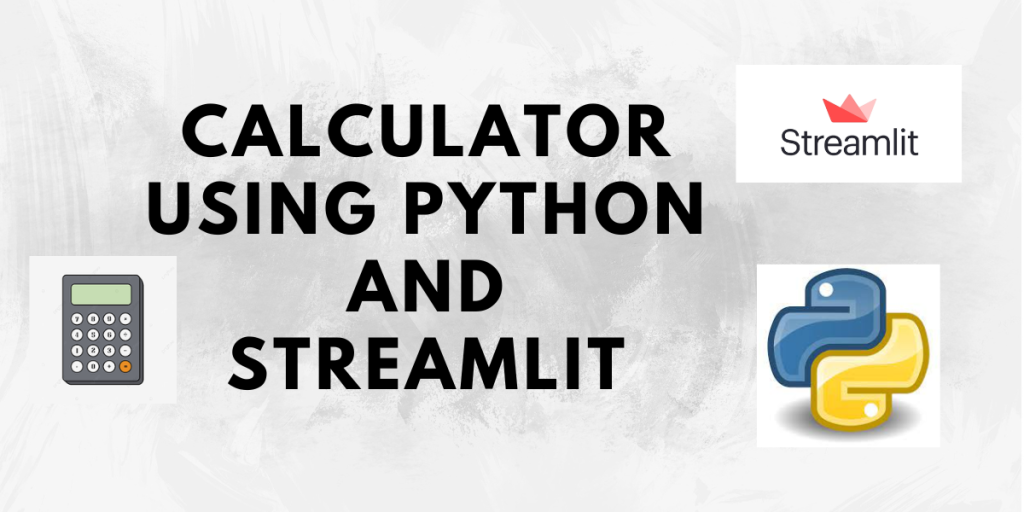# Calculator App using Streamlit – Easy Step-by-Step MethodHello and welcome to this tutorial! This is the third tutorial in the Streamlit tutorial series. So far in the first two tutorials, we have had an introduction to Streamlit and learned how to install Streamlit, create a ‘Hello World’ app, text elements, and widgets available in Streamlit, and also how to style our app by using Theming in Streamlit.

We highly recommend you go through the following tutorials if you haven’t already:

## Creating a Calculator App using Streamlit

Now that we know all of this, we must practice what we have learned before moving forward. So, in this tutorial, we will develop a simple calculator application by applying the knowledge of the concepts we have learned now. So let us begin!

### Step 1: Create the basic app

Create a file Python file in your favorite IDE. You can name it anything. Here, the file name is `calculator.py`.

```import streamlit as st

st.title("Calculator App using Streamlit")

# creates a horizontal line
st.write("---")
```

Here we have first just imported Streamlit and added a title to our app. Also, below the title, we have added a horizontal line in order to separate the title from the below section.
Remember that we can run the app using the command:

```streamlit run calculator.py
```

The basic app looks like this:

### Step 2: Accept user input

Since we are building a calculator we want our user to be able to enter the numbers to perform operations on. Let’s do this.

```# input 1
num1 = st.number_input(label="Enter first number")

# input 2
num2 = st.number_input(label="Enter second number")
```

As we want to perform operations on numbers, we have used the `numeric_input()` input widget. You can learn more about it here. So we have created two input fields `num1` and `num2` that we will be using.

### Step 3: Add mathematical operations

Now that we have the input, let us add the operations that the user can perform on it. Since we want to perform only one operation at a time on the inputs, we will radio buttons to provide the options, as radio buttons allow only one selection at a time.

```st.write("Operation")

operation = st.radio("Select an operation to perform:",
```

The radio button has a label and an options list that lists out all the operations that we can possibly allow the user to perform. This list is not exhaustive and can be customized as per your requirements.

### Step 4: Add the functionality to the calculator

We have the inputs as well as the operations. Now we need to define how both of these will work together. We shall create a function named `calculate` that performs the operation according to the input and operation selected by the user.

```ans = 0

def calculate():
ans = num1 + num2
elif operation == "Subtract":
ans = num1 - num2
elif operation == "Multiply":
ans = num1 * num2
elif operation=="Divide" and num2!=0:
ans = num1 / num2
else:
st.warning("Division by 0 error. Please enter a non-zero number.")
ans = "Not defined"

```

We have first created a variable `ans` to store the result. The `calculate` function just checks which operation was selected by the user from the options list and performs the action accordingly.

One thing to note is that division by zero is not defined. So when the Divide operation is selected, we need to first check if the second input is 0. If so, give a warning message that this conveys that this operation is not possible, otherwise just perform the division.

After the successful completion of the operation, we display the result using a success status element from Streamlit.

### Step 5: Add the calculate button

To see the logic written in the above step in action, we need a calculate button. So let us add it.

```if st.button("Calculate result"):
calculate()
```

When the button is clicked, the answer will be calculated and displayed on the screen.

## Complete Code for Building a Calculator in Streamlit

```import streamlit as st

st.title("Calculator App using Streamlit")

# creates a horizontal line
st.write("---")

# input 1
num1 = st.number_input(label="Enter first number")

# input 2
num2 = st.number_input(label="Enter second number")

st.write("Operation")

operation = st.radio("Select an operation to perform:",

ans = 0

def calculate():
ans = num1 + num2
elif operation == "Subtract":
ans = num1 - num2
elif operation == "Multiply":
ans = num1 * num2
elif operation=="Divide" and num2!=0:
ans = num1 / num2
else:
st.warning("Division by 0 error. Please enter a non-zero number.")
ans = "Not defined"

if st.button("Calculate result"):
calculate()
```

## Playing around with our calculator app

Let us now give some input and see the results.